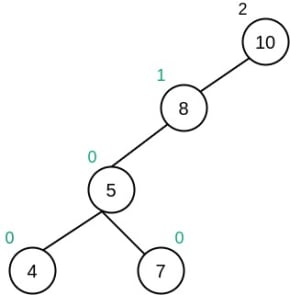Home Data Structures Introduction to AVL Tree and its properties

# Introduction to AVL Tree and its properties

AVL tree(Adelson-Velsky and Landis tree named after it’s inventor) is a height-balanced Binary Search Tree such that the difference between the height of a left subtree and a right subtree (of a node) is 1, 0 or -1. It has the following properties:

1. It should be a Binary Search Tree i.e, key in the left subtree are always less than or equal to the root, and key in right subtree should be greater than root.
2. The difference between the hight of left subtree and right subtree is known as balance factors and its value should always be 1, 0, -1.
3. The left and right subtrees should also be AVL trees.
##### Balance Factor

Balance Factor is the difference between the height of the left subtree and the right subtree and its value should be -1, 0, or 1.

BalanceFactor = height(left subtree) – height(right subtree)

BalanceFactor ∈ {-1, 0, 1}

##### ExampleNot a valid AVL tree because the balance factor of node 10 is 2.

### The time complexity of various operations on an AVL tree

###### Worst Case
Height O(log N) O(log N)
Search O(log N) O(log N)
Insert O(log N) O(log N)
Delete O(log N) O(log N)
Tree Traversal O(N) O(N)

The reason AVL is better than BST is that most of its operation takes O(log N) time in worst case too as compared to BST where in worst-case time complexity becomes O(N), where N is the number of nodes in the tree.

##### Height

The maximum height of an AVL tree is floor(log2 N) can’t exceed 1.44*log2N, where N is the number of nodes in the given tree.

Given the height of an AVL tree as h, the maximum number of nodes it can have is  2h+1 – 1 and the minimum number of nodes it can have is given by the formula:
N(h) = N(h-1) + N(h-2) + 1 for n>2 where N(0) = 1 and N(1) = 2.